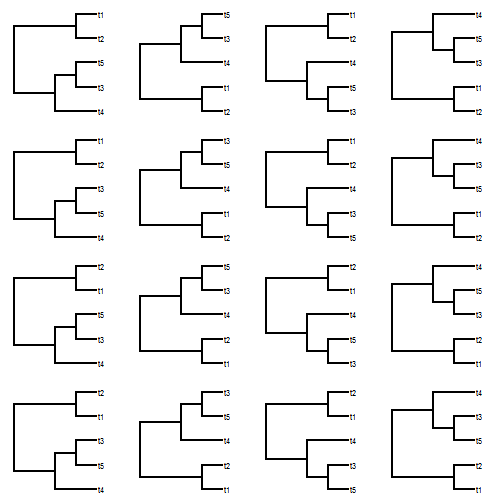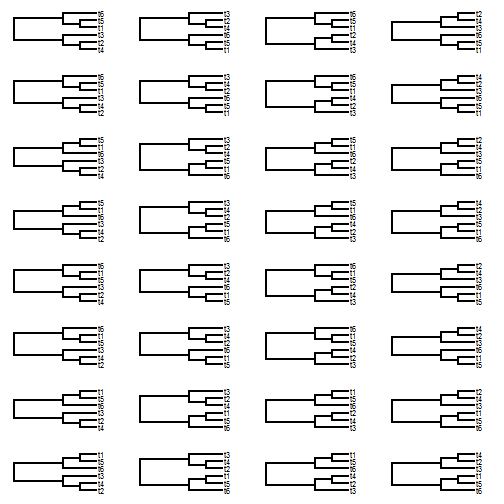## Saturday, August 27, 2016

### Function to find all possible rotations of the nodes of a tree

The following is a function to find all possible rotated topologies for a bifurcating tree. Note that the number of such trees is very large! In particular, it should be 2m for m internal nodes. This is the same as N - 1 for N if the tree is perfectly bifurcating.

This quantity is thus:

``````2^(5-1) ## for five taxa
``````
``````##  16
``````
``````2^(8-1) ## for eight taxa
``````
``````##  128
``````
``````2^(10-1) ## for 10 taxa
``````
``````##  512
``````
``````2^(20-1) ## for twenty taxa
``````
``````##  524288
``````
``````2^(100-1) ## for 100 taxa
``````
``````##  6.338253e+29
``````

You get the idea.

Obviously, we should only do this for relatively small trees!

Here is the function:

``````library(phytools)
## function to compute all rotations
allRotations<-function(tree){
if(!is.binary.tree(tree)){
was.binary<-FALSE
if(is.null(tree\$edge.length)){
tree<-compute.brlen(tree)
tree<-multi2di(tree)
} else was.binary<-TRUE
nodes<-1:tree\$Nnode+Ntip(tree)
trees<-vector(mode="list",length=2^length(nodes))
ii<-2
trees[]<-tree
for(i in 1:length(nodes)){
N<-ii-1
for(j in 1:N){
trees[[ii]]<-rotate(trees[[j]],nodes[i])
ii<-ii+1
}
}
if(!was.binary){
trees<-lapply(trees,di2multi)
function(x){
x\$edge.length<-NULL
x
})
}
class(trees)<-"multiPhylo"
trees
}
``````

Let's try it:

``````## five taxa
tree<-rtree(n=5,br=NULL)
trees<-allRotations(tree)
trees
``````
``````## 16 phylogenetic trees
``````
``````par(mfrow=c(4,4))
plotTree(trees,mar=rep(1.1,4))
````````````## six taxa
trees<-allRotations(rtree(n=6,br=NULL))
trees
``````
``````## 32 phylogenetic trees
``````
``````par(mfrow=c(8,4))
plotTree(trees,mar=rep(1.1,4),ylim=c(0.8,6.2))
````````````## ten taxa
trees<-allRotations(rtree(n=10,br=NULL))
par(mfrow=c(32,16))
plotTree(trees,lwd=1,fsize=0.1)
``````